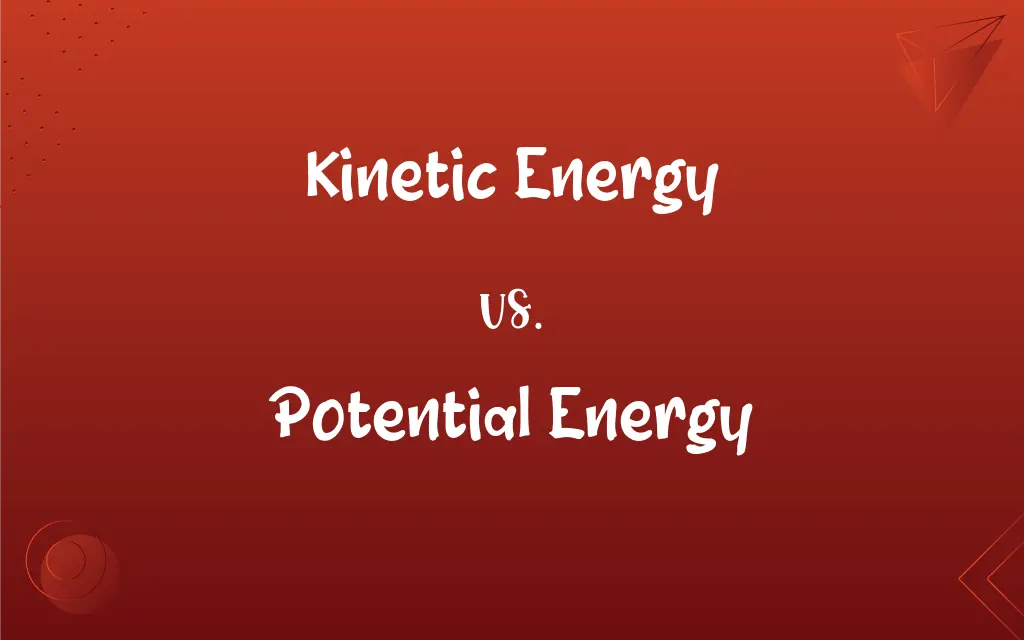# Kinetic Energy vs. Potential Energy: What's the Difference?Edited by Huma Saeed || By Sumera Saeed || Updated on October 16, 2023
Kinetic energy is energy in motion, while potential energy is stored energy due to position or state.## Key Differences

Kinetic energy is the energy an object possesses due to its motion, dependent on both its mass and velocity, whereas potential energy is the energy stored within an object due to its position, condition, or configuration.
Kinetic energy is observed when an object is in motion; for instance, a rolling ball or a moving car, while potential energy exists in a stationary object awaiting release, such as water behind a dam or a rock perched at the edge of a cliff.
The calculation of kinetic energy is straightforward, using the object's mass and the square of its velocity, while potential energy's calculation depends on factors like position relative to other objects, height, and gravity or inherent structural stresses.
Kinetic energy is directly transferrable, as seen in collisions or momentum transfer, whereas potential energy requires a change in the object's state or position for the energy to be released or converted.
Both kinetic and potential energy are forms of mechanical energy, with the former representing active energy in motion and the latter indicative of dormant energy that has the potential for motion.

## Comparison Chart

### State

Active, in motion
Dormant, due to position or state

### Calculation

Based on mass and velocity
Based on position, condition, or stress

### Transfer

Directly in events like collisions
Requires change in state or position

### Representation

Energy of motion
Energy of position

### Dependency

Velocity and mass
Height, gravity, structural stresses

## Kinetic Energy and Potential Energy Definitions

#### Kinetic Energy

Dependent on velocity and mass.
Even a small object can have huge kinetic energy if it moves fast enough.

#### Potential Energy

Can transform into kinetic energy.
When the ball rolls down, its potential energy turns into kinetic energy.

#### Kinetic Energy

Transferrable through collisions or momentum.
During the collision, kinetic energy was transferred from one car to the other.

#### Potential Energy

A dormant form of energy.
Chemicals stored in batteries have potential energy that can be used later.

#### Kinetic Energy

The active energy in moving objects.
The kinetic energy of the molecules increased with the temperature.

#### Potential Energy

Energy held by an object because of its position.
A book on a shelf has potential energy due to gravity.

#### Kinetic Energy

A measurable quantity of motion.
As the car accelerated, it gained more kinetic energy.

#### Potential Energy

Related to the position or state of an object.
Water behind a dam contains massive potential energy.

#### Kinetic Energy

Energy that a body possesses by virtue of being in motion.
The ball, when thrown, carries kinetic energy.

#### Potential Energy

Stored energy ready to be converted into other forms.
The stretched spring holds potential energy.

## FAQs

#### What is kinetic energy?

Kinetic energy is the energy an object possesses due to its motion.

#### What defines potential energy?

Potential energy is the energy stored in an object due to its position or state.

#### Does kinetic energy increase with speed?

Yes, kinetic energy increases with the square of the object's speed.

#### What factors affect potential energy?

Factors like height, mass, gravity, and structural configuration affect potential energy.

#### What's a common example of potential energy?

A common example is a stretched or compressed spring.

#### How is kinetic energy calculated?

Kinetic energy is calculated based on an object's mass and the square of its velocity.

#### Can potential energy be converted to other forms?

Yes, potential energy can convert into kinetic energy or other energy forms when conditions change.

#### Is kinetic energy always conserved?

In an ideal, closed system, yes, but it can be transferred or dissipated in real-world scenarios.

#### Can potential energy be found in everyday life?

Yes, objects at a height, stretched materials, and charged batteries all contain potential energy.

#### Does all moving object have kinetic energy?

Yes, any object in motion possesses kinetic energy.

#### Can we feel kinetic energy?

Not directly, but we can feel the effects of kinetic energy, like wind produced by a moving object.

#### Is potential energy dependent on height?

Yes, gravitational potential energy is directly proportional to height.

#### How does kinetic energy relate to work?

Work is the process of transferring energy, and when work is done to accelerate an object, it gains kinetic energy.

#### Is potential energy a static form of energy?

Yes, it's considered static until it's converted to kinetic energy or another form of energy.

#### Is kinetic energy scalar or vector?

Kinetic energy is a scalar quantity; it has magnitude but no direction.

#### What happens to potential energy when an object falls?

It converts to kinetic energy as the object accelerates downward.

#### Can kinetic energy be negative?

No, kinetic energy cannot be negative as it's dependent on mass and the square of velocity, both positive.

#### How does mass impact potential energy?

Greater mass increases gravitational potential energy when positioned above ground level.

#### Does potential energy relate to stability?

Yes, objects with lower potential energy are typically more stable than those with higher potential energy.

#### What unit is kinetic energy measured in?

Kinetic energy is measured in joules (J).